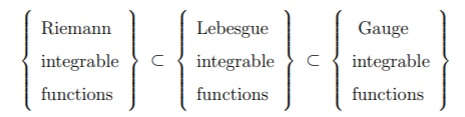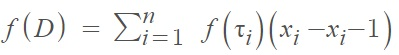# Gauge Integral

A gauge integral is a generalization of the Riemann integral, to include a much wider class of functions. The basic idea behind the gauge integral is to modify the standard delta-epsilon definition of the Riemann integral by replacing the constant δ with a function, γ, called a gauge. A gauge function is basically a positive function δ(x) defined on a finite interval [a, b].

A gauge represents an open interval of variable length . In other words, while Riemann integration is concerned with the summation of uniformly fine partitions (i.e. very thin rectangles of equal width seen in Riemann sums), Gauge integration is concerned with locally fine partitions that can vary from point to point. The gauge function &gamm; (τi) defines this locally fine partition.

The Gauge integral goes by many other names, including the Denjoy-Perron, generalized Riemann, Henstock, Henstock-Kurzweil, HK, and Kurzweil integral.

## Why The Gauge Integral was Developed

The Riemann integral and Lebesgue integral cannot be used to integrate all functions. In fact, only a very small class of functions is Riemann integrable . In addition, these two types of integration fail to provide good convergence theorems even for integrable functions. A classic example of where Riemann integration fails is with 1Q, representing the characteristic function of the rational numbers over a certain interval. Although rationality dictates that this integral should be zero, 1Q isn’t Riemann integrable anywhere. Many other functions in applied mathematics and physics show this “bad” behavior, which renders Riemann integration impractical outside of elementary calculus studies.

Although other integrals have been formulated to “fix” these issues, including the Lebesgue integral, these are complicated and largely inaccessible to the general calculus student. The gauge integral addresses these issues and is also fairly easy to understand. The definition is only slightly different from the definition of the Riemann integral , yet Gauge integration includes all functions that are Riemann integrable or Lebesgue integrable.## From Riemann to Gauge Integrals

As the gauge integral is an extension of the Riemann integral, it may be helpful to review some properties of Riemann integrals.

Let’s say you wanted to integrate a function f over an interval [a, b]. The first step is to form a partition of [a, b] with subintervals [xi−1, xi] by choosing a series of numbers xi so that

a = x0 < x1 < x2 < … < xn = b.

Next, choose a number τ (called a tag) to create a tagged partition, D, from each interval so that:
D : a = x0 < x1 < x2 < … < xn = b, τi ∈ [xi−1, xi].

The Riemann sum for this interval [a, b] is:The size of the interval associated with a certain tag is determined by the function γ, which can be shown as follows. Using the behavior of f at τ, we can assign a neighborhood to it γ(τ). This results in an interval-valued function γ defined on [a, b]. Now consider the sums you get from those tagged partitions where [xi−1, xi] ⊆ γ(τi). A tagged partition is γ-fine when:
[xi−1, xi] ⊆ γ(τi), for all i = 1, 2,…,n.

With a Riemann sum, you usually choose the partition first, then you choose the associated tags afterward. A major difference with gauge integration is that you have to think of the tags as fixed, then you decide if xi−1 and xi are close enough to τi to make f(τi)(xi, xi−1) a good approximation.

Much of the above overview was based on McInnis’s paper on Gauge Integration. You can download the PDF, which has more detail and a few examples, here: Gauge Integration

## References

 McInnis, E. (2002). Gauge Integration. Retrieved August 5, 2021 from: https://calhoun.nps.edu/bitstream/handle/10945/4857/02Sep_McInnis.pdf?sequence=1&isAllowed=y
 Herschlag, G. A Brief Introduction to Gauge Integration. Retrieved August 5, 2021 from: http://math.uchicago.edu/~may/VIGRE/VIGRE2006/PAPERS/Herschlag.pdf
 Schechter, E. (2009). An Introduction to The Gauge Integral. Retrieved August 5, 2021 from: https://math.vanderbilt.edu/schectex/ccc/gauge/

CITE THIS AS:
Stephanie Glen. "Gauge Integral" From StatisticsHowTo.com: Elementary Statistics for the rest of us! https://www.statisticshowto.com/gauge-integral/